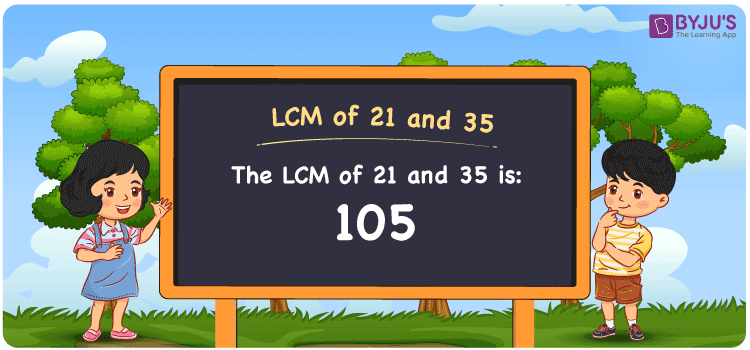Checkout JEE MAINS 2022 Question Paper Analysis : Checkout JEE MAINS 2022 Question Paper Analysis :

# LCM of 21 and 35

LCM of 21 and 35 is 105. The value of LCM is the number evenly divisible by the given two numbers. Least common multiple of 21 and 35 is the number which is the smallest obtained from the common multiples. (21, 42, 63, 84, 105, 126, ….) and (35, 70, 105, 140, 175,….) are the multiples of 21 and 35. The LCM can be easily determined using methods such as prime factorisation, division and by listing the multiples.

## What is LCM of 21 and 35?

The answer to this question is 105. The LCM of 21 and 35 using various methods is shown in this article for your reference. The LCM of two non-zero integers, 21 and 35, is the smallest positive integer 105 which is divisible by both 21 and 35 with no remainder.## How to Find LCM of 21 and 35?

LCM of 21 and 35 can be found using three methods:

• Prime Factorisation
• Division method
• Listing the multiples

### LCM of 21 and 35 Using Prime Factorisation Method

The prime factorisation of 21 and 35, respectively, is given by:

21 = 3 x 7 = 3¹ x 7¹

35 = 5 x 7 = 5¹ x 7¹

LCM (21, 35) = 105

### LCM of 21 and 35 Using Division Method

We’ll divide the numbers (21, 35) by their prime factors to get the LCM of 21 and 35 using the division method (preferably common). The LCM of 21 and 35 is calculated by multiplying these divisors.

 5 21 35 3 21 7 7 7 7 x 1 1

No further division can be done.

Hence, LCM (21, 35) = 105

### LCM of 21 and 35 Using Listing the Multiples

To calculate the LCM of 21 and 35 by listing out the common multiples, list the multiples as shown below.

 Multiples of 21 Multiples of 35 21 35 42 70 63 105 84 140 105 175

The smallest common multiple of 21 and 35 is 105.

Therefore LCM (21, 35) = 105

## Video Lesson on Applications of LCM## LCM of 21 and 35 Solved Examples

What is the LCM if the GCD is 7 and the product of two numbers is 735?

Solution:

It is given that

Product of two numbers = 735

GCD = 7

We know that

LCM x GCD = Product of two numbers

LCM = Product/GCD

LCM = 735/7

LCM = 105

Hence, the LCM is 105.

## Frequently Asked Questions on LCM of 21 and 35

### What is the LCM of 21 and 35?

The LCM of 21 and 35 is 105. In order to get the LCM value, you need to find the multiples of 21 and 35 and the multiple which is the smallest and divisible by 21 and 35 has to be found.

### What are the methods used to calculate the LCM of 21 and 35?

The methods used to calculate the LCM of 21 and 35 are Prime Factorization Method, Division Method and Listing multiples.

### Calculate the GCF if the LCM of 21 and 35 is 105.

LCM x GCF = 21 x 35

Given

LCM of 21 and 35 = 105

105 x GCF = 735

GCF = 735/105 = 7